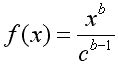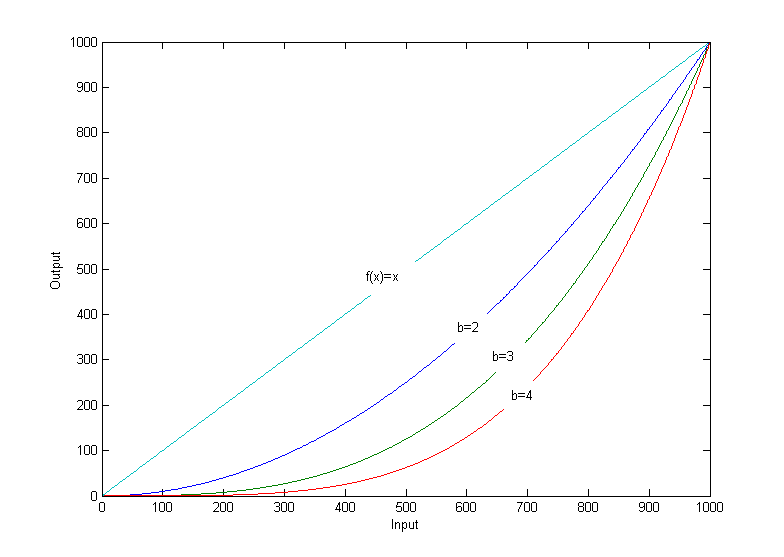# RyanDoyle.net

home posts contact

# Bias Random Numbers

01 Sep 2008

I recently needed to find a function that would be able to bias random numbers. Out of a set of 1000 random numbers, I wanted more of these to be smaller instead of true random numbers (or as true as random number generators are). I had a look at simple parabolic and exponential functions and eventially devised the following equation.Where b = factor of bias and c = max. integer of random function. The higher value of b, the more biased the function will be towards lower numbers. c is defined as when f(x) = x. As stated previously, c is the highest possible integer of the random function you are using. If your RNG is generating a maximum number of 1000, then c = 1000.

Shown is a plot of several b values